International
Tables for
Crystallography
Volume D
Physical properties of crystals
Edited by A. Authier

International Tables for Crystallography (2006). Vol. D, ch. 1.1, pp. 24-25

## Section 1.1.4.10.3. Reduction of the number of independent components of third-rank polar tensors due to the symmetry of the strain and stress tensors

A. Authiera*

aInstitut de Minéralogie et de la Physique des Milieux Condensés, Bâtiment 7, 140 rue de Lourmel, 75015 Paris, France
Correspondence e-mail: aauthier@wanadoo.fr

#### 1.1.4.10.3. Reduction of the number of independent components of third-rank polar tensors due to the symmetry of the strain and stress tensors

| top | pdf |

Equation (1.1.4.5)can be written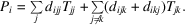The sums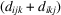forhave a definite physical meaning, but it is impossible to devise an experiment that permits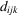and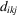to be measured separately. It is therefore usual to set them equal: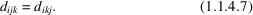It was seen in Section 1.1.4.8.1that the components of a third-rank tensor can be represented as a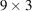matrix which can be subdivided into threesubmatrices: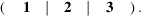Relation (1.1.4.7)shows that submatrices 1 and 2 are identical.

One puts, introducing a two-index notation,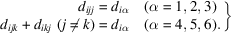Relation (1.1.4.7)becomes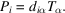The coefficients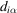may be written as a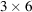matrix: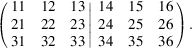This matrix is constituted by twosubmatrices. The left-hand one is identical to the submatrix 1, and the right-hand one is equal to the sum of the two submatrices 2 and 3: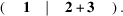The inverse piezoelectric effect expresses the strain in a crystal submitted to an applied electric field: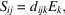where the matrix associated with the coefficientsis amatrix which is the transpose of that of the coefficients used in equation (1.1.4.5), as shown in Section 1.1.1.4.

The components of the Voigt strain matrix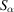are then given by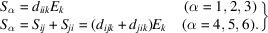This relation can be written simply as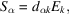where the matrix of the coefficients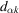is a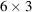matrix which is the transpose of thematrix.

There is another set of piezoelectric constants (see Section 1.1.5) which relates the stress,, and the electric field,, which are both intensive parameters: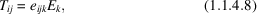where a new piezoelectric tensor is introduced,. Its components can be represented as a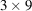matrix: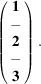Both sides of relation (1.1.4.8)remain unchanged if the indices i and j are interchanged, on account of the symmetry of the stress tensor. This shows that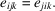Submatrices 2 and 3 are equal. One introduces here a two-index notation through the relation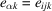, and the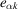matrix can be written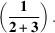The relation between the full and the reduced matrix is therefore different for theand thetensors. This is due to the particular property of the strain Voigt matrix (1.1.4.6), and as a consequence the relations between nonzero components of the reduced matrices are different for certain point groups (3, 32,,,).